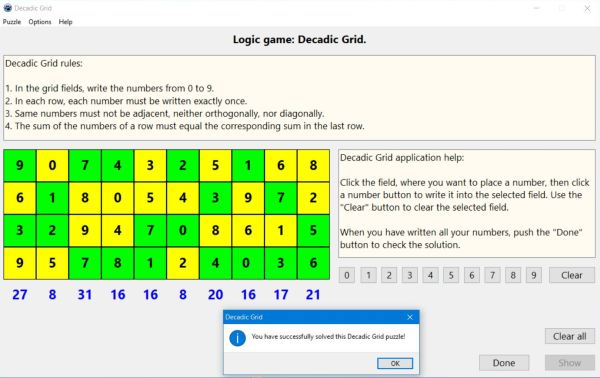# Computing: Free Pascal Programming

## Logic number puzzle: Decadic Grid.

Description: Two difficulty levels puzzle game with numbers to be placed onto a grid. The rules of the game are as follows:

1. In the grid fields, write the numbers from 0 to 9.
2. In each row, each number must be written exactly once.
3. Same numbers must not be adjacent, neither orthogonally, nor diagonally.
4. The sum of the numbers of a row must equal the corresponding sum in the last row.
The game is a typical logic game: In order to find the correct solution of the puzzle, you have to explore the numbers in a row and the column sums, and by logical thinking (concluding from what you know, that a given field must be a given number, or on the contrary, can't be a given number), determine, which number has to be written into which field.
The game is played exclusively with the mouse: Click the field, where you want to write a number, then click one of the 10 number buttons to write the corresponding number or Clear to remove the number from the actually selected field.

Issue: The generation of the 6x10 grid puzzle may take some time. No real issue, but I'm sure, that it would be possible to optimize the code in order to get new puzzles started without any delay.

Free Pascal features: Changing the color of shapes and the caption of static-texts during runtime. Using buttons to insert characters into a given control's caption. Two-dimensional arrays (classic Pascal).

Screenshot: